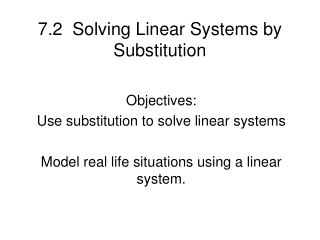DownloadDownload Presentation7.2 Solving Linear Systems by Substitution

# 7.2 Solving Linear Systems by Substitution

Download Presentation## 7.2 Solving Linear Systems by Substitution

- - - - - - - - - - - - - - - - - - - - - - - - - - - E N D - - - - - - - - - - - - - - - - - - - - - - - - - - -
##### Presentation Transcript

1. 7.2 Solving Linear Systems bySubstitution Objectives: Use substitution to solve linear systems Model real life situations using a linear system.

2. Solve one of the equations for one of its variables. • Substitute the expression from Step 1 into the other equation and solve for the other variable. • Substitute the value from Step 2 into the revised equation Step and solve. • Check the solution in each of the original equations.

3. SOLVE THE LINEAR SYSTEM -X + Y = 1 Rearrange equation so 2X + Y = -2 you have X = or Y = Y = X + 1 Replace Y with X + 1 in second equation 2X + X + 1 = -2 Solve for X 3X + 1 = -2 3X = -3 X = -1 Substitute -1 in for X in one • (-1) + Y = 1 of the original equations. 1 + Y = 1 Solve for Y. The point of Y = 0 intersection is X = -1 and Y = 0

4. Solve the system. -x + y = 1 x + 2y = 4 2x + y = -2 -x + y = -7 2x + 2y = 3 2x + 6y = 15 X – 4y = -1 x = 2y

5. An office supply company sells two types of fax machines. They charge \$150 for one of the machines and \$225 for the other. If the company sold 22 fax machines for a total of \$3900 last month, how many of each type were sold?

6. One day at the softball tournament \$1590 was collected from the 321 people admitted to the game. The price of each adult admission is \$6 and the student admission is \$4. How many adults and how many children were admitted that day?

7. WARM UP TO ALGEBRA!!!! A. SOLVE FOR Y. 2X + 4Y = -2 B. SOLVE FOR X. 2Y – X = -7 • FIND THE SLOPE OF THE LINE THAT GOES THROUGH THE POINTS (0, 8) AND (8, 0).